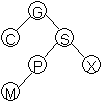Exercises
Binary Search Trees

1. The binary search tree provides us with a structure that allows us O(    ) access to any node in the structure - an improvement over the sequential search of a(n)      (list which is O(n).)

2. A binary tree is a structure in which each node is capable of having      successor nodes, called     . The unique starting node is called the     .

3. A      node is one that has no children. A node of a binary tree is itself the root of a smaller tree called a     . All nodes appearing in a subtree are called      of the root node of the tree - conversely, the root node is called an      of all nodes appearing below it. The root node of a tree is said to have level     . The maximum level in a tree determines its      and the level contains at most      nodes.

4. In a binary search tree, what is true of all nodes in the right subtree of some given node?

5. If you want to get ride of an existing tree, the statement `tree = NULL` is not recommended. Why?

6. Any node inserted in a binary search tree necessarily comes a      node.

7. Is the recursive function Find on page 410 a good use of recursion? Why or why not?

8. Minimizing the height of a Binary Search Tree will maximize

9. What three cases are considered in developing the Remove function?

10. Name the three BST traversals.

11. Given the character input ( M G B H S P F C), draw the BST and state the tree traversals.

12. In destroying a tree which traversal should be used?

13. Which BST traversal lists the info fields of the tree nodes in sorted fashion?

Consider the tree below.14. How many subtrees are shown?

15. Insert nodes containing T and N. Redraw the tree.

16. After part 15, the trees height is   ?

17. Name the ancestors of P.

18. Interchange all subtrees with their corresponding right subtrees by redrawing the tree again.

Continue to:  Unit 8 / Prev / Next